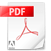## Chapter 4: Equation of state1. Why "equation of state"
211229 Mineral Physics I 4 Equation of s4.1a.pdf2. Birch-Murnaghan equation of state
211229 Mineral Physics I 4. Equation ofKatsura & Tange 2019.pdfKatsura & Tange 2020 in Japanese.pdf3. Vinet equation of state
211229 Mineral Physics I 4. Equation of4.3a.pdf4. Murnaghan's equation of state
211229 Mineral Physics I 4. Equation of4.4a.pdf5. Examination of Birch-Murnaghan equation of state
211229 Mineral Physics I 4. Equation ofKatsura & Tange 2019.pdfKatsura & Tange 2020 in Japanese.pdf6. Thermal equation of state
220124 Mineral Physics I 4 Equation of4.6a.pdf4.6b.pdf7. Mie-Grüneisen-Debye equation of state
220123 Mineral Physics I 4 Equation of